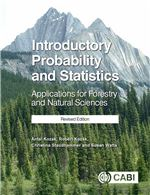Cookies on CAB eBooks

Like most websites we use cookies. This is to ensure that we give you the best experience possible.

Continuing to use www.cabi.org  means you agree to our use of cookies. If you would like to, you can learn more about the cookies we use.

CAB eBooks

Ebooks on agriculture and the applied life sciences from CAB International

CABI Book Info

Introductory probability and statistics: applications for forestry and natural sciences.Description

This book with 15 chapters utilizes basic descriptive statistics and probability, as well as commonly used statistical inferential tools to introduce topics that are commonplace in a forestry context such as hypothesis texting, design of experiments, sampling methods, non-parametric tests and statistical quality control. It also contains examples and exercises drawn from the fields of forestry, wood science, and conservation.

Metrics

Book Chapters

 Chapter: 1 (Page no: 1) Statistics and data: what do numbers have to do with trees? Author(s): Kozak, A. Kozak, R. A. Staudhammer, C. L. Watts, S. B. Chapter: 2 (Page no: 9) Descriptive statistics: making sense of data. Author(s): Kozak, A. Kozak, R. A. Staudhammer, C. L. Watts, S. B. Chapter: 3 (Page no: 35) Probability: the foundation of statistics. Author(s): Kozak, A. Kozak, R. A. Staudhammer, C. L. Watts, S. B. Chapter: 4 (Page no: 61) Random variables and probability distributions: outcomes of random experiments. Author(s): Kozak, A. Kozak, R. A. Staudhammer, C. L. Watts, S. B. Chapter: 5 (Page no: 79) Some discrete probability distributions: describing data that are counted. Author(s): Kozak, A. Kozak, R. A. Staudhammer, C. L. Watts, S. B. Chapter: 6 (Page no: 93) Continuous distributions and the normal distribution: describing data that are measured. Author(s): Kozak, A. Kozak, R. A. Staudhammer, C. L. Watts, S. B. Chapter: 7 (Page no: 111) Sampling distributions: the foundation of inference. Author(s): Kozak, A. Kozak, R. A. Staudhammer, C. L. Watts, S. B. Chapter: 8 (Page no: 147) Estimation: determining the value of population parameters. Author(s): Kozak, A. Kozak, R. A. Staudhammer, C. L. Watts, S. B. Chapter: 9 (Page no: 173) Tests of hypotheses: making claims about population parameters. Author(s): Kozak, A. Kozak, R. A. Staudhammer, C. L. Watts, S. B. Chapter: 10 (Page no: 201) Goodness-of-fit and test for independence: testing distributions. Author(s): Kozak, A. Kozak, R. A. Staudhammer, C. L. Watts, S. B. Chapter: 11 (Page no: 217) Regression and correlation: relationships between variables. Author(s): Kozak, A. Kozak, R. A. Staudhammer, C. L. Watts, S. B. Chapter: 12 (Page no: 251) Analysis of variance: testing differences between several means. Author(s): Kozak, A. Kozak, R. A. Staudhammer, C. L. Watts, S. B. Chapter: 13 (Page no: 277) Sampling methods and design of experiments: collecting data. Author(s): Kozak, A. Kozak, R. A. Staudhammer, C. L. Watts, S. B. Chapter: 14 (Page no: 287) Non-parametric tests: testing when distributions are unknown. Author(s): Kozak, A. Kozak, R. A. Staudhammer, C. L. Watts, S. B. Chapter: 15 (Page no: 305) Quality control: statistics for production and processing. Author(s): Kozak, A. Kozak, R. A. Staudhammer, C. L. Watts, S. B.

Book details

• Author Affiliation
• Faculty of Forestry, The University of British Columbia, Vancouver, BC, Canada.
• Year of Publication
• 2019
• ISBN
• 9781789243307
• Record Number
• 20193360983